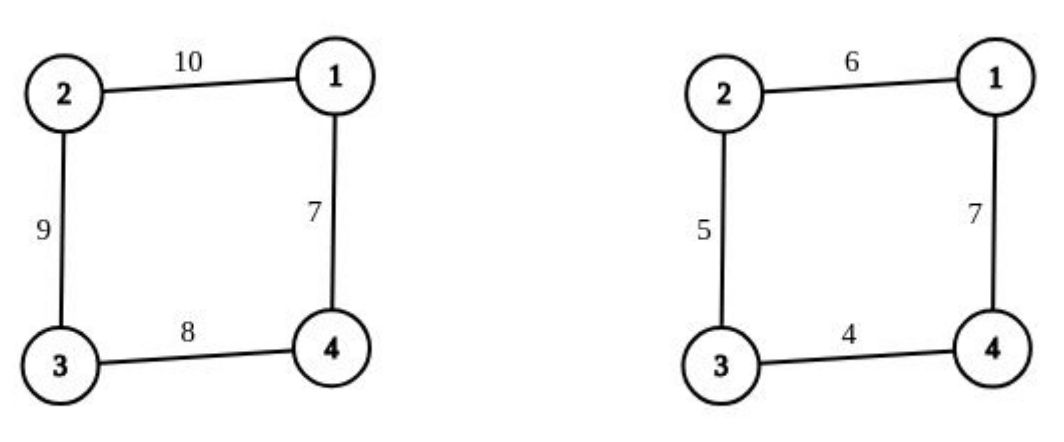시간 제한 메모리 제한 제출 정답 맞은 사람 정답 비율
1 초 512 MB 2 2 2 100.000%

문제

"In the input there is an integer number N. Find the Nth digit of the number 0.135791113151719 ..."

In order to succeed in the next attempt to pass the exam and so saving himself from repeating the academic year, he decided to practice by being the main character in tasks such as the following:

An undirected graph of N nodes and M edges is given. Each edge has a value, an integer number less than 109.

A simple cycle (a cycle without repeating nodes) is good if the bitwise XOR of all the values of the cycle’s edges is equal to zero.

Ivan can make a number of operations on the graph. An operation consists of the following steps:

• Ivan selects an integer number x;
• then he selects a non-empty subset of edges of the given graph;
• and then he applies bitwise XOR by number x on all the the selected edges (If one of the selected edges has a value p, after the described operation, the new value of that edge will be equal to p XOR x)

Ivan wants to obtain a graph in which every simple cycle is good. Also, he wants to do so in as few operations as possible. Determine the minimum possible number of operations after which each simple cycle is good and print them. It can be proved that it is always possible to meet Ivan's requirements with a certain sequence of operations.

입력

The first line contains two positive integers N and M (1 ≤ N, M ≤ 100 000), the number of nodes and the number of edges.

In the ith of the following M lines there is a description of the ith edge: three integer numbers ai, bi i pi (1 ≤ ai, bi ≤ N, ai ≠ bi, 0 ≤ pi ≤ 109), the nodes connected with the ith edge and the value of the edge. The graph is connected and all the edges are different.

출력

In the first line of output, print K, minimum number of task operations.

In each of the following K lines, print a sequence numbers separated by space:

• the first number in the row is the number x from the operation;
• the second number is B, the number of selected bridges;
• then follows B numbers, Ei (1 ≤ Ei ≤ M) which indicate labels of the selected edges in the ascending order.

All numbers in the output should be less than or equal to 2·109.

예제 입력 1

4 4
1 2 10
2 3 9
3 4 8
4 1 7


예제 출력 1

1
12 3 1 2 3


예제 입력 2

2 1
1 2 3


예제 출력 2

0


예제 입력 3

6 8
1 2 6
2 3 1
3 5 6
3 1 5
4 5 0
3 6 0
4 2 8
4 1 1


예제 출력 3

2
2 2 4 6
15 1 7


힌트

Explanation of test samples:

In the first test sample, the initial graph is given in the image left below, and the final graph (after applying XOR on the first three edges with 12) is given in the image right below. The only cycle in the graph is good because XOR of its edges is 0.

In the second test sample, there is no cycle, so the claim "every simple cycle is good" is trivially fulfilled. That is why the number of required operations is 0.출처

• 스페셜 저지를 만든 사람: koosaga